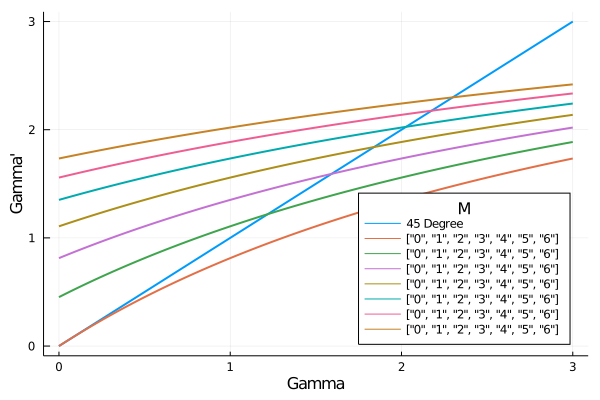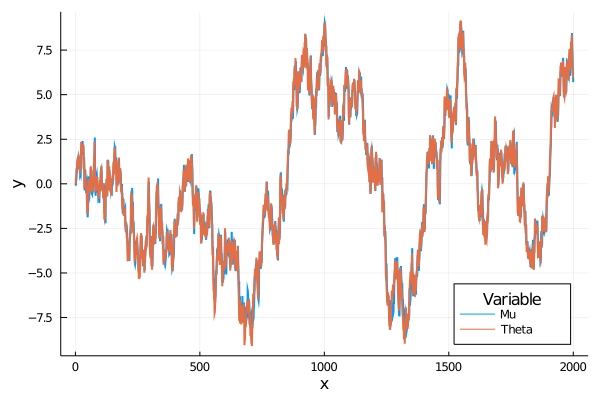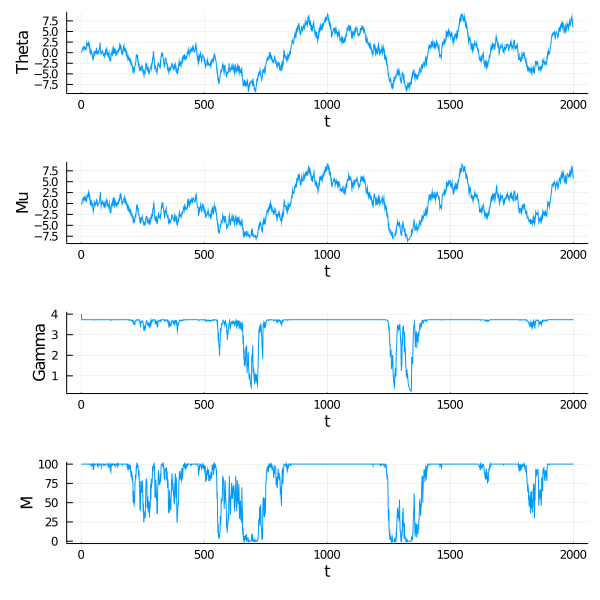• Lectures
• Code
• Notebooks
• Community

# Uncertainty Traps¶

## Overview¶

In this lecture we study a simplified version of an uncertainty traps model of Fajgelbaum, Schaal and Taschereau-Dumouchel [FSTD15].

The model features self-reinforcing uncertainty that has big impacts on economic activity.

In the model,

• Fundamentals vary stochastically and are not fully observable.
• At any moment there are both active and inactive entrepreneurs; only active entrepreneurs produce.
• Agents – active and inactive entrepreuneurs – have beliefs about the fundamentals expressed as probability distributions.
• Greater uncertainty means greater dispersions of these distributions.
• Entrepreneurs are risk averse and hence less inclined to be active when uncertainty is high.
• The output of active entrepreneurs is observable, supplying a noisy signal that helps everyone inside the model infer fundamentals.
• Entrepreneurs update their beliefs about fundamentals using Bayes’ Law, implemented via Kalman filtering.

Uncertainty traps emerge because:

• High uncertainty discourages entrepreneurs from becoming active.
• A low level of participation – i.e., a smaller number of active entrepreneurs – diminishes the flow of information about fundamentals.
• Less information translates to higher uncertainty, further discouraging entrepreneurs from choosing to be active, and so on.

Uncertainty traps stem from a positive externality: high aggregate economic activity levels generates valuable information.

## The Model¶

The original model described in [FSTD15] has many interesting moving parts.

Here we examine a simplified version that nonetheless captures many of the key ideas.

### Fundamentals¶

The evolution of the fundamental process $\{\theta_t\}$ is given by

$$\theta_{t+1} = \rho \theta_t + \sigma_{\theta} w_{t+1}$$

where

• $\sigma_\theta > 0$ and $0 < \rho < 1$
• $\{w_t\}$ is IID and standard normal

The random variable $\theta_t$ is not observable at any time.

### Output¶

There is a total $\bar M$ of risk averse entrepreneurs.

Output of the $m$-th entrepreneur, conditional on being active in the market at time $t$, is equal to

$$x_m = \theta + \epsilon_m \quad \text{where} \quad \epsilon_m \sim N \left(0, \gamma_x^{-1} \right) \tag{1}$$

Here the time subscript has been dropped to simplify notation.

The inverse of the shock variance, $\gamma_x$, is called the shock’s precision.

The higher is the precision, the more informative $x_m$ is about the fundamental.

Output shocks are independent across time and firms.

### Information and Beliefs¶

All entrepreneurs start with identical beliefs about $\theta_0$.

Signals are publicly observable and hence all agents have identical beliefs always.

Dropping time subscripts, beliefs for current $\theta$ are represented by the normal distribution $N(\mu, \gamma^{-1})$.

Here $\gamma$ is the precision of beliefs; its inverse is the degree of uncertainty.

These parameters are updated by Kalman filtering.

Let

• $\mathbb M \subset \{1, \ldots, \bar M\}$ denote the set of currently active firms
• $M := |\mathbb M|$ denote the number of currently active firms
• $X$ be the average output $\frac{1}{M} \sum_{m \in \mathbb M} x_m$ of the active firms

With this notation and primes for next period values, we can write the updating of the mean and precision via

$$\mu' = \rho \frac{\gamma \mu + M \gamma_x X}{\gamma + M \gamma_x} \tag{2}$$

$$\gamma' = \left( \frac{\rho^2}{\gamma + M \gamma_x} + \sigma_\theta^2 \right)^{-1} \tag{3}$$

These are standard Kalman filtering results applied to the current setting.

Exercise 1 provides more details on how (2) and (3) are derived, and then asks you to fill in remaining steps.

The next figure plots the law of motion for the precision in (3) as a 45 degree diagram, with one curve for each $M \in \{0, \ldots, 6\}$.

The other parameter values are $\rho = 0.99, \gamma_x = 0.5, \sigma_\theta =0.5$Points where the curves hit the 45 degree lines are long run steady states for precision for different values of $M$.

Thus, if one of these values for $M$ remains fixed, a corresponding steady state is the equilibrium level of precision

• high values of $M$ correspond to greater information about the fundamental, and hence more precision in steady state
• low values of $M$ correspond to less information and more uncertainty in steady state

In practice, as we’ll see, the number of active firms fluctuates stochastically.

### Participation¶

Omitting time subscripts once more, entrepreneurs enter the market in the current period if

$$\mathbb E [ u(x_m - F_m) ] > c \tag{4}$$

Here

• the mathematical expectation of $x_m$ is based on (1) and beliefs $N(\mu, \gamma^{-1})$ for $\theta$
• $F_m$ is a stochastic but previsible fixed cost, independent across time and firms
• $c$ is a constant reflecting opportunity costs

The statement that $F_m$ is previsible means that it is realized at the start of the period and treated as a constant in (4).

The utility function has the constant absolute risk aversion form

$$u(x) = \frac{1}{a} \left(1 - \exp(-a x) \right) \tag{5}$$

where $a$ is a positive parameter.

Combining (4) and (5), entrepreneur $m$ participates in the market (or is said to be active) when

$$\frac{1}{a} \left\{ 1 - \mathbb E [ \exp \left( -a (\theta + \epsilon_m - F_m) \right) ] \right\} > c$$

Using standard formulas for expectations of lognormal random variables, this is equivalent to the condition

$$\psi(\mu, \gamma, F_m) := \frac{1}{a} \left( 1 - \exp \left( -a \mu + a F_m + \frac{a^2 \left( \frac{1}{\gamma} + \frac{1}{\gamma_x} \right)}{2} \right) \right) - c > 0 \tag{6}$$

## Implementation¶

We want to simulate this economy.

We’ll want a named tuple generator of the kind that we’ve seen before.

And we need methods to update $\theta$, $\mu$ and $\gamma$, as well as to determine the number of active firms and their outputs.

The updating methods follow the laws of motion for $\theta$, $\mu$ and $\gamma$ given above.

The method to evaluate the number of active firms generates $F_1, \ldots, F_{\bar M}$ and tests condition (6) for each firm.

### Setup¶

In :
using InstantiateFromURL
# optionally add arguments to force installation: instantiate = true, precompile = true
github_project("QuantEcon/quantecon-notebooks-julia", version = "0.8.0")

In :
using LinearAlgebra, Statistics
using DataFrames, Parameters, Plots
gr(fmt = :png);

In :
UncertaintyTrapEcon = @with_kw (a = 1.5, # risk aversion
γ_x = 0.5, # production shock precision
ρ = 0.99, # correlation coefficient for θ
σ_θ = 0.5, # standard dev. of θ shock
num_firms = 100, # number of firms
σ_F = 1.5, # standard dev. of fixed costs
c = -420.0, # external opportunity cost
μ_init = 0.0, # initial value for μ
γ_init = 4.0, # initial value for γ
θ_init = 0.0, # initial value for θ
σ_x = sqrt(a / γ_x)) # standard dev. of shock

Out:
##NamedTuple_kw#253 (generic function with 2 methods)

In the results below we use this code to simulate time series for the major variables.

## Results¶

Let’s look first at the dynamics of $\mu$, which the agents use to track $\theta$We see that $\mu$ tracks $\theta$ well when there are sufficient firms in the market.

However, there are times when $\mu$ tracks $\theta$ poorly due to insufficient information.

These are episodes where the uncertainty traps take hold.

During these episodes

• precision is low and uncertainty is high
• few firms are in the market

To get a clearer idea of the dynamics, let’s look at all the main time series at once, for a given set of shocksNotice how the traps only take hold after a sequence of bad draws for the fundamental.

Thus, the model gives us a propagation mechanism that maps bad random draws into long downturns in economic activity.

## Exercises¶

### Exercise 1¶

Fill in the details behind (2) and (3) based on the following standard result (see, e.g., p. 24 of [YS05]).

Fact Let $\mathbf x = (x_1, \ldots, x_M)$ be a vector of IID draws from common distribution $N(\theta, 1/\gamma_x)$ and let $\bar x$ be the sample mean. If $\gamma_x$ is known and the prior for $\theta$ is $N(\mu, 1/\gamma)$, then the posterior distribution of $\theta$ given $\mathbf x$ is

$$\pi(\theta \,|\, \mathbf x) = N(\mu_0, 1/\gamma_0)$$

where

$$\mu_0 = \frac{\mu \gamma + M \bar x \gamma_x}{\gamma + M \gamma_x} \quad \text{and} \quad \gamma_0 = \gamma + M \gamma_x$$

### Exercise 2¶

Modulo randomness, replicate the simulation figures shown above

• Use the parameter values listed as defaults in the function UncertaintyTrapEcon.

## Solutions¶

### Exercise 1¶

This exercise asked you to validate the laws of motion for $\gamma$ and $\mu$ given in the lecture, based on the stated result about Bayesian updating in a scalar Gaussian setting.

The stated result tells us that after observing average output $X$ of the $M$ firms, our posterior beliefs will be

$$N(\mu_0, 1/\gamma_0)$$

where

$$\mu_0 = \frac{\mu \gamma + M X \gamma_x}{\gamma + M \gamma_x} \quad \text{and} \quad \gamma_0 = \gamma + M \gamma_x$$

If we take a random variable $\theta$ with this distribution and then evaluate the distribution of $\rho \theta + \sigma_\theta w$ where $w$ is independent and standard normal, we get the expressions for $\mu'$ and $\gamma'$ given in the lecture.

## Exercise 2¶

First let’s replicate the plot that illustrates the law of motion for precision, which is

$$\gamma_{t+1} = \left( \frac{\rho^2}{\gamma_t + M \gamma_x} + \sigma_\theta^2 \right)^{-1}$$

Here $M$ is the number of active firms. The next figure plots $\gamma_{t+1}$ against $\gamma_t$ on a 45 degree diagram for different values of $M$

In :
econ = UncertaintyTrapEcon()
@unpack ρ, σ_θ, γ_x = econ # simplify names

# grid for γ and γ_{t+1}
γ = range(1e-10, 3, length = 200)
M_range = 0:6
γp = 1 ./ (ρ^2 ./ (γ .+ γ_x .* M_range') .+ σ_θ^2)

labels = ["0", "1", "2", "3", "4", "5", "6"]

plot(γ, γ, lw = 2, label = "45 Degree")
plot!(γ, γp, lw = 2, label = labels)
plot!(xlabel = "Gamma", ylabel = "Gamma'", legend_title = "M", legend = :bottomright)

Out:The points where the curves hit the 45 degree lines are the long run steady states corresponding to each $M$, if that value of $M$ was to remain fixed. As the number of firms falls, so does the long run steady state of precision.

Next let’s generate time series for beliefs and the aggregates – that is, the number of active firms and average output

In :
function simulate(uc, capT = 2_000)
# unpack parameters
@unpack a, γ_x, ρ, σ_θ, num_firms, σ_F, c, μ_init, γ_init, θ_init, σ_x = uc

# draw standard normal shocks
w_shocks = randn(capT)

# aggregate functions
# auxiliary function ψ
function ψ(γ, μ, F)
temp1 = -a * (μ - F)
temp2 = 0.5 * a^2 / (γ + γ_x)
return (1 - exp(temp1 + temp2)) / a - c
end

# compute X, M
function gen_aggregates(γ, μ, θ)
F_vals = σ_F * randn(num_firms)
M = sum(ψ.(Ref(γ), Ref(μ), F_vals) .> 0) # counts number of active firms
if any(ψ(γ, μ, f) > 0 for f in F_vals) # ∃ an active firm
x_vals = θ .+ σ_x * randn(M)
X = mean(x_vals)
else
X = 0.0
end
return (X = X, M = M)
end

# initialize dataframe
X_init, M_init = gen_aggregates(γ_init, μ_init, θ_init)
df = DataFrame(γ = γ_init, μ = μ_init, θ = θ_init, X = X_init, M = M_init)

# update dataframe
for t in 2:capT
# unpack old variables
θ_old, γ_old, μ_old, X_old, M_old = (df.θ[end], df.γ[end], df.μ[end], df.X[end], df.M[end])

# define new beliefs
θ = ρ * θ_old + σ_θ * w_shocks[t-1]
μ = (ρ * (γ_old * μ_old + M_old * γ_x * X_old))/(γ_old + M_old * γ_x)
γ = 1 / (ρ^2 / (γ_old + M_old * γ_x) + σ_θ^2)

# compute new aggregates
X, M = gen_aggregates(γ, μ, θ)
push!(df, (γ = γ, μ = μ, θ = θ, X = X, M = M))
end

# return
return df
end

Out:
simulate (generic function with 2 methods)

First let’s see how well $\mu$ tracks $\theta$ in these simulations

In :
df = simulate(econ)

plot(eachindex(df.μ), df.μ, lw = 2, label = "Mu")
plot!(eachindex(df.θ), df.θ, lw = 2, label = "Theta")
plot!(xlabel = "x", ylabel = "y", legend_title = "Variable", legend = :bottomright)

Out:Now let’s plot the whole thing together

In :
len = eachindex(df.θ)
yvals = [df.θ, df.μ, df.γ, df.M]
vars = ["Theta", "Mu", "Gamma", "M"]

plt = plot(layout = (4,1), size = (600, 600))

for i in 1:4
plot!(plt[i], len, yvals[i], xlabel = "t", ylabel = vars[i], label = "")
end

plot(plt)

Out:• Share page